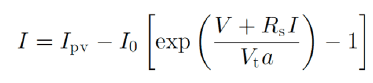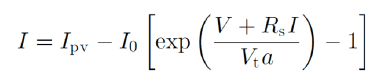# Solving Diode Equation for Current?

• physics_197

## Homework Statement

I need to find the current, but not sure how to rearrange the equation because of the I in the exp.

## Homework Equations## The Attempt at a Solution

Thanks, just need to be pointed in the right direction. Seems like I will either be in ln or exp.

Also, just a follow up question, the diode ideality constant... Is there a way to solve for it? My notes just say its between 1 - 1.5, Should I just assume its 1? The manufacturers data sheet does not mention a value for this.

## Homework Statement

I need to find the current, but not sure how to rearrange the equation because of the I in the exp.

## Homework Equations## The Attempt at a Solution

Thanks, just need to be pointed in the right direction. Seems like I will either be in ln or exp.
Because I appears on its own and as the argment of the exponential, you can't solve for it using elementary operations.

Also, just a follow up question, the diode ideality constant... Is there a way to solve for it? My notes just say its between 1 - 1.5, Should I just assume its 1? The manufacturers data sheet does not mention a value for this.
Are you talking about I0? That one you can solve for.

No, I'm talking about just I. So how would I go about finding it?

No, I'm talking about just I. So how would I go about finding it?
Using numerical methods

Thank you, I used Newtons method on matlab, I think it should be correct :)

Also, do any of you have an idea about the diode ideality factor? I am trying to model a PV module in Matlab and the data sheet does not mention this. I read online that people tend to assume its just 1... Any ideas?

Nevermind! After running through the code using different values (between 1 and 2), There is no difference in terms of the current... well either, it can be neglected or my code is wrong (I tried entering a large number, that affected the current)

Thank you, I used Newtons method on matlab, I think it should be correct :)

Also, do any of you have an idea about the diode ideality factor? I am trying to model a PV module in Matlab and the data sheet does not mention this. I read online that people tend to assume its just 1... Any ideas?
Which variable is the diode ideality factor? That's what I was asking about in post #4.